# Clustering

## What's clustering?

• Clustering, in its broadest form, is figure out how to group data
• It's generally a form of unsupervised learning. Which is to say that you don't know a priori which data belong to which groups.
• It's often used in
• Text mining to find topics
• Image recognition, image segmentation, brain region segmentation
• Spike sorting
• Bioinformatics, gene families
• Marketing: Shopping habits, trends, recommendations
• Anomaly detection

## Types of clustering

• Combinatorial algorithms - work directly on the observed data with no direct reference to an underlying probability model
• Mixture modeling - supposes that the data is an i.i.d sample from some population described by a probability density function
• Mode seekers - "bump hunters" take a nonparametric perspective, attempting to directly estimate distinct modes of the probability density function
Source: The Elements of Statistical Learning

## Combinatorial clustering

$k$-means
Agglomerative clustering

### $k$-means

1. Assign points to a cluster
2. For each cluster, calculate it's mean center point
3. Assign points to a cluster based on the closest center
4. Repeat steps 2-3 until no point changes cluster

### $k$-means

kmeansStart(stuff, 2) kmeansStop()
kmeansStart(stuff, 3) kmeansStop()
kmeansStart(stuff, 4) kmeansStop()
kmeansStart(stuff, 5) kmeansStop()

### Problems with k-means

• Dependent on initial conditions
• Only linear boundaries
• Clusters tend to be around the same size
• What if cluster is inside another?
• How do you know how many clusters to use?
• Outliers? Use $k$-medoids

Agglomerative single-linkage clustering is one of several "bottom-up" types of hierarchical clustering.
2. Compute the distances of each cluster
• In this case, find the two closest points between clusters
• In other cases you take the average distance of points between clusters
• In complete-linkage you find the furthest points between clusters
3. Merge the two clusters with the minimal distance
4. Repeat steps 2 and 3 until you have the desired number of clusters

agglo.kmeansStart(2) agglo.kmeansStop()
agglo.agglomerativeStart(2, 100) agglo.agglomerativeStop()
agglo.agglomerativeStart(4, 60) agglo.agglomerativeStop()

• Naive implementation is $O(n^3)$ slow
• Exhibits "chaining". Points in the same cluster can be further away from each other than from a point in another cluster.
• Again, how do you know how many clusters to use?
• Alternatives?
• Also, spectral clustering

### Agglomerative

agglo2.kmeansStart(2) agglo2.kmeansStop() $k$-means
agglo2.agglomerativeStart(4, 100, "single") agglo2.agglomerativeStop() Single linkage
agglo2.agglomerativeStart(4, 100, "complete") agglo2.agglomerativeStop() Complete linkage
agglo2.agglomerativeStart(4, 100, "average") agglo2.agglomerativeStop() Average linkage

## The importance of representational space

### RGB Space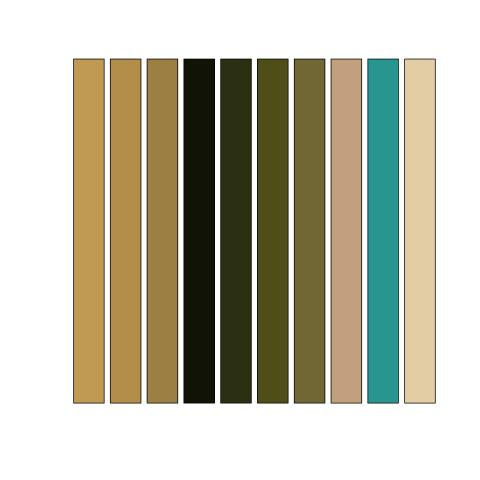### HSV Space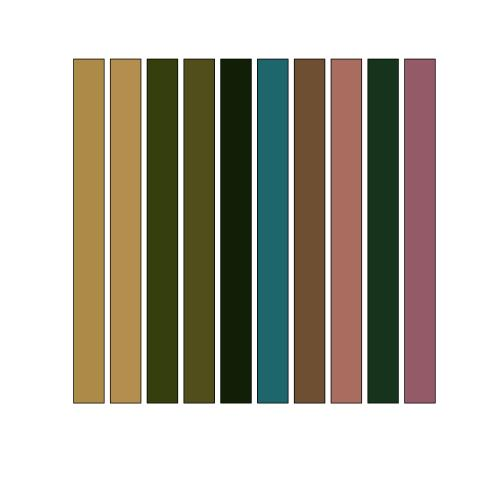### LAB Space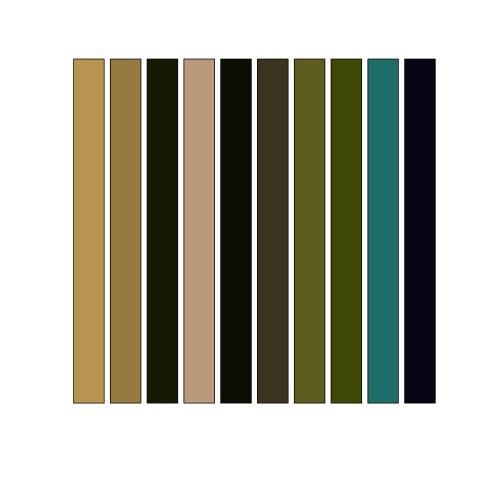## Mixture Modeling

### How would you fit this?https://mollermara.com/blog/em-algorithm/

#### $k$-means doesn't do too well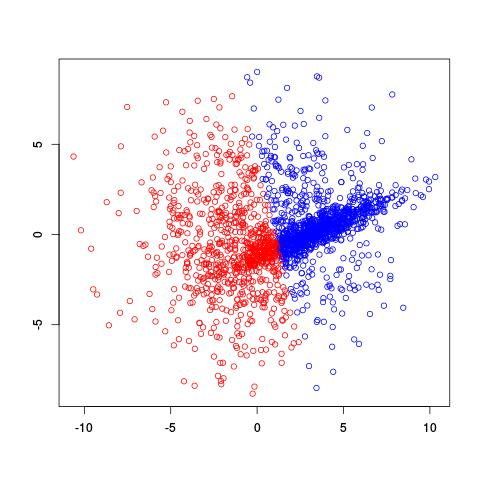#### Use Gaussian mixture modeling!

$p(x) = \sum_{i=1}^k \alpha_i \cdot \text{N}(x|\mu_i, \sigma^2_i)$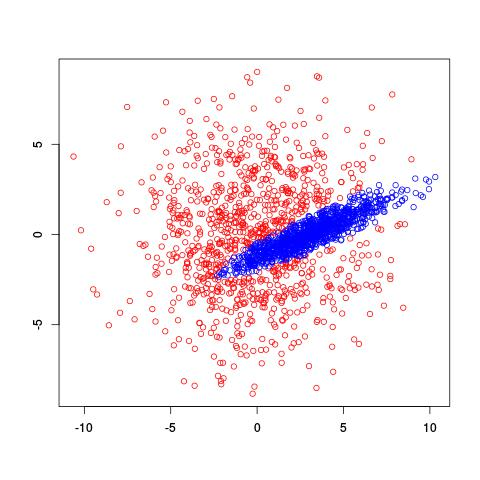#### Expectation-Maximization algorithm

• Gaussian mixture models are fit similarly to $k$-means
• But you assign points a probability for each mixture, instead of outright assigning. This is called the expectation step
• In the maximization step, you take all the points and their cluster-probabilities and find the best-fitting Gaussian for each cluster. You estimate both the mean and the covariance

### Example EM-iteration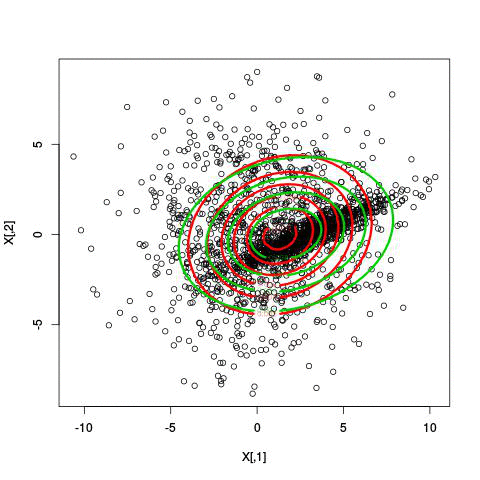### Comments on the EM algorithm

• Like $k$-means, it's sensitive to initial conditions
• However, you get not just an assignment but the probability a point is in each cluster
• You can use the likelihood with a model scoring mechanism like AIC or BIC to count the number of clusters
• Mixture models, however, can also be fit using Markov-Chain Monte Carlo methods like Gibbs sampling

#### Fitting different numbers of clusters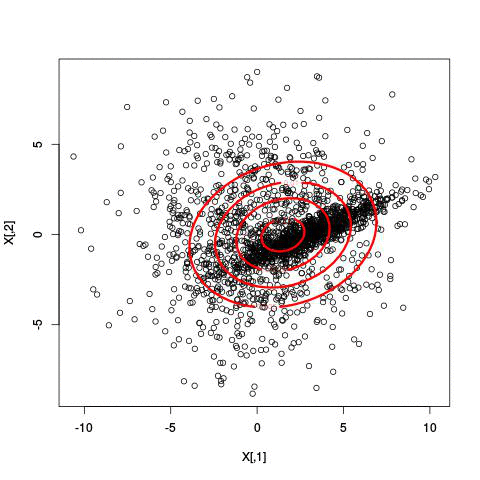#### Latent Dirichlet Allocation for topic modeling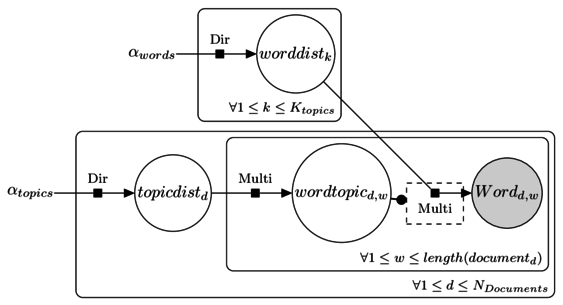#### LDA topic modeling in JAGS

model {
for (k in 1 : Ktopics ) {
worddist[k,1:Nwords] ~ ddirch(alphaWords)
}
for( d in 1 : Ndocs ) {
topicdist[d,1:Ktopics] ~ ddirch(alphaTopics)
for (w in 1 : length[d]) {
wordtopic[d,w] ~ dcat(topicdist[d,1:Ktopics])
word[d,w] ~ dcat(worddist[wordtopic[d,w],1:Nwords])
}
}
}


#### LDA topic modeling in JAGS (cont)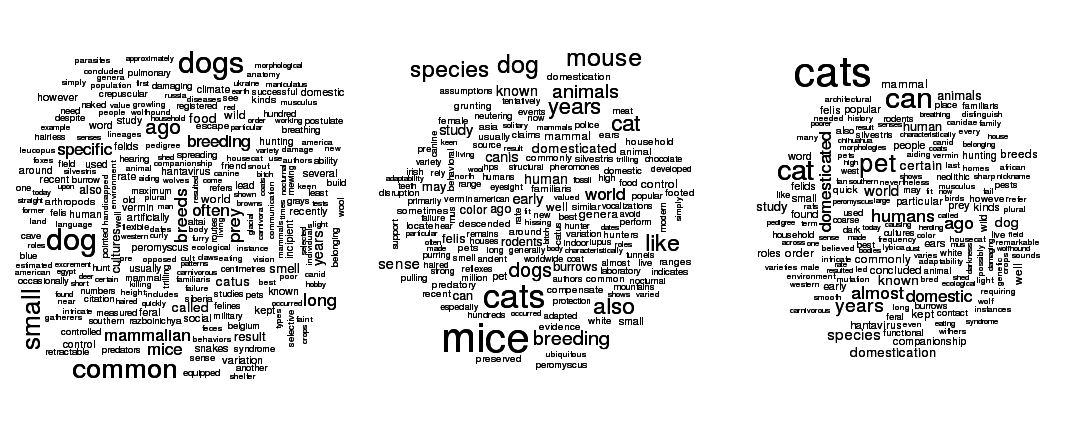An example of LDA run on Wikipedia articles on cats, dogs, and mice.

## Wrapping Up

• Which clustering algorithm you use depends on your assumptions
• Central to clustering is the idea of distance. What space you use or how you define distance have a great impact on what clusters you get.